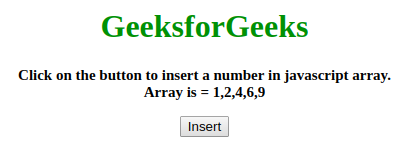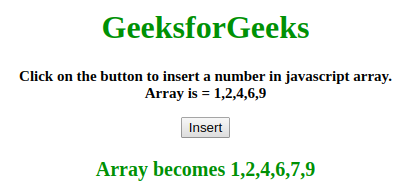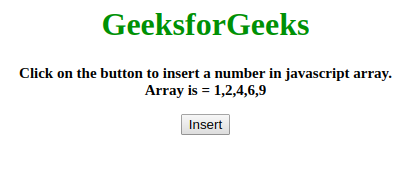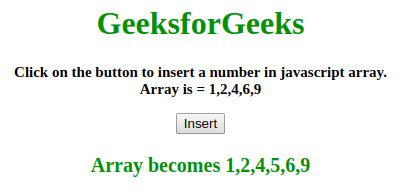# What is the Efficient way to insert a number into a sorted array of numbers in JavaScript?

Given an array of numbers and the task is to insert a number into the sorted array using JavaScript. There are many approaches to solve this problem two of them are given below:

Approach 1:

• First take the values in a variable (lets arr).
• Make sure it is sorted.
• In this example, the complexity is O(n), where n is the number of elements in the array available.
• The method findLoc is searching for the element just greater than the element that we want to insert.
• Method return the index of the location.
• perform the insert operation using .splice() method.

Example: This example illustrate the approach discussed above.

 ` ` `<``html``> ` ` `  `<``head``> ` ` ` ` `  `<``body` `style``=``"text-align:center;"` `id``=``"body"``> ` `    ``<``h1` `style``=``"color:green;"``>  ` `      ``GeeksforGeeks  ` `    `` ` `    ``<``p` `id``=``"GFG_UP"`  `       ``style``=``"font-size: 15px; font-weight: bold;"``> ` `    `` ` `    ``<``button` `onclick``=``"gfg_Run()"``> ` `        ``Insert ` `    `` ` `    ``<``p` `id``=``"GFG_DOWN"`  `       ``style``=``"color:green; font-size: 20px; font-weight: bold;"``> ` `    `` ` `    ``<``script``> ` `        ``var el_up = document.getElementById("GFG_UP"); ` `        ``var el_down = document.getElementById("GFG_DOWN"); ` `        ``var today = new Date(); ` `        ``var arr = [1, 2, 4, 6, 9]; ` `        ``el_up.innerHTML = "Click on the button to insert a number "+ ` `                       ``"in javascript array.<``br``> Array is = " + arr; ` ` `  `        ``function add(el, arr) { ` `            ``arr.splice(findLoc(el, arr) + 1, 0, el); ` `            ``return arr; ` `        ``} ` ` `  `        ``function findLoc(el, arr, st, en) { ` `            ``st = st || 0; ` `            ``en = en || arr.length; ` `            ``for (i = 0; i < ``arr.length``; i++) { ` `                ``if (arr[i] > el) ` `                    ``return i - 1; ` `            ``} ` `            ``return en; ` `        ``} ` ` `  `        ``function gfg_Run() { ` `            ``add(7, arr); ` `            ``el_down.innerHTML = "Array becomes " + arr; ` `        ``} ` `    `` ` ` ` ` `  ` `

Output:

• Before clicking on the button:• After clicking on the button:Approach 2:

• In this example, the complexity is O(Logn), where n is the number of elements in the array.
• A method findLoc is searching for the location where the element should be present.
• Method return the index of the location by using binary search algorithm.
• perform the insert operation using .splice() method.

Example: This example illustrate the approach discussed above.

 ` ` `<``html``> ` ` `  `<``head``> ` ` ` ` `  `<``body` `style``=``"text-align:center;"` `id``=``"body"``> ` `    ``<``h1` `style``=``"color:green;"``>   ` `      ``GeeksforGeeks   ` `    `` ` `    ``<``p` `id``=``"GFG_UP"`  `       ``style``=``"font-size: 15px; font-weight: bold;"``> ` `    `` ` `    ``<``button` `onclick``=``"gfg_Run()"``> ` `        ``Insert ` `    `` ` `    ``<``p` `id``=``"GFG_DOWN"`  `       ``style``=``"color:green; font-size: 20px; font-weight: bold;"``> ` `    `` ` `    ``<``script``> ` `        ``var el_up = document.getElementById("GFG_UP"); ` `        ``var el_down = document.getElementById("GFG_DOWN"); ` `        ``var today = new Date(); ` `        ``var arr = [1, 2, 4, 6, 9]; ` `        ``el_up.innerHTML = "Click on the button to insert a "+ ` `          ``"number in javascript array.<``br``> Array is = " + arr; ` ` `  `        ``function add(el, arr) { ` `            ``arr.splice(findLoc(el, arr) + 1, 0, el); ` `            ``return arr; ` `        ``} ` ` `  `        ``function findLoc(el, arr, st, en) { ` `            ``st = st || 0; ` `            ``en = en || arr.length; ` `            ``var pivot = parseInt(st + (en - st) / 2, 10); ` `            ``if (en - st <= 1 || arr[pivot] === el) return pivot; ` `            ``if (arr[pivot] < ``el``) { ` `                ``return findLoc(el, arr, pivot, en); ` `            ``} else { ` `                ``return findLoc(el, arr, st, pivot); ` `            ``} ` `        ``} ` ` `  `        ``function gfg_Run() { ` `            ``add(5, arr); ` `            ``el_down.innerHTML` `= ``"Array becomes "` `+ arr; ` `        ``} ` `    `` ` ` ` ` `  ` `

Output:

• Before clicking on the button:• After clicking on the button:My Personal Notes arrow_drop_upCheck out this Author's contributed articles.

If you like GeeksforGeeks and would like to contribute, you can also write an article using contribute.geeksforgeeks.org or mail your article to contribute@geeksforgeeks.org. See your article appearing on the GeeksforGeeks main page and help other Geeks.

Please Improve this article if you find anything incorrect by clicking on the "Improve Article" button below.